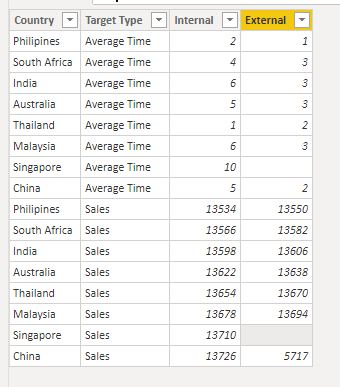cancel
Showing results for
Did you mean:Helper I

## Conditional Format on Column Chart - Multiple locations

Hoping to get some insight on solving this problem.

1. See attached layout of multiple column charts on a page.  I have two such pages : Average Time and Sales.

The value on the chart is obtained from a calculated measure. Also take note the the slicer to display "Internal" and "External" value.2.  This is a summary of what I am trying to achive.

• The target for each country will be maintained in a shaporeint table. The layout of this table is not rigid. Please feel free to suggest a suitable layout. For explanation, an example is shown herewith.I am hoping to find a solution where the color of the bar changes to either red or green based on whether the target was hot or miss.

Thanks in advance and much appreciated.

2 ACCEPTED SOLUTIONSCommunity Support

Hi @dearears ,

Create a table using below dax expression:

``````Table 2 = ADDCOLUMNS(CROSSJOIN(VALUES('Table'[Country]),UNION(ROW("Target Type","Average Time"),ROW("Target Type","Sales"))),"Internal",
SWITCH(TRUE(),
[Target Type]="Average Time",CALCULATE(AVERAGE('Table'[Time(days)]),FILTER('Table','Table'[Group]="Internal"&&'Table'[Country]=EARLIER('Table'[Country]))),
[Target Type]="Sales",CALCULATE(SUM('Table'[Sales ('000)]),FILTER('Table','Table'[Group]="Internal"&&'Table'[Country]=EARLIER('Table'[Country])))),
"External",
SWITCH(TRUE(),
[Target Type]="Average Time",CALCULATE(AVERAGE('Table'[Time(days)]),FILTER('Table','Table'[Group]="External"&&'Table'[Country]=EARLIER('Table'[Country]))),
[Target Type]="Sales",CALCULATE(SUM('Table'[Sales ('000)]),FILTER('Table','Table'[Group]="External"&&'Table'[Country]=EARLIER('Table'[Country])))))

``````

And you will see:For the related .pbix file,pls see attached.

Best Regards,
KellyHelper I

Many thanks Kelly. Your solution partially answered my question and I could tweak it a little to suit my needs.

4 REPLIES 4Community Support

Hi @dearears ,

Could you pls provide a monthly data of each country for test?

Best Regards,
KellyHelper I

Trust this will help.Community Support

Hi @dearears ,

Create a table using below dax expression:

``````Table 2 = ADDCOLUMNS(CROSSJOIN(VALUES('Table'[Country]),UNION(ROW("Target Type","Average Time"),ROW("Target Type","Sales"))),"Internal",
SWITCH(TRUE(),
[Target Type]="Average Time",CALCULATE(AVERAGE('Table'[Time(days)]),FILTER('Table','Table'[Group]="Internal"&&'Table'[Country]=EARLIER('Table'[Country]))),
[Target Type]="Sales",CALCULATE(SUM('Table'[Sales ('000)]),FILTER('Table','Table'[Group]="Internal"&&'Table'[Country]=EARLIER('Table'[Country])))),
"External",
SWITCH(TRUE(),
[Target Type]="Average Time",CALCULATE(AVERAGE('Table'[Time(days)]),FILTER('Table','Table'[Group]="External"&&'Table'[Country]=EARLIER('Table'[Country]))),
[Target Type]="Sales",CALCULATE(SUM('Table'[Sales ('000)]),FILTER('Table','Table'[Group]="External"&&'Table'[Country]=EARLIER('Table'[Country])))))

``````

And you will see:For the related .pbix file,pls see attached.

Best Regards,
KellyHelper I

Many thanks Kelly. Your solution partially answered my question and I could tweak it a little to suit my needs.